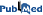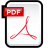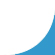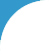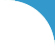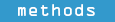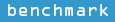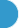ContrastRank
A new method for tumor samples classification

 Definitions and Assumptions We assume that rare variants are more likely to have functional effect than common variants and among the rare variants the non-synonymous single nucleotide variants (nsSNVs) have the strongest impact. This assumption is supported by the analysis of annotated variants in dbSNP (Sherry, et al., 2001), which shows that the fraction of annotated pathogenic variants is significantly higher for nsSNVs . Moreover, among the nsSNVs, the rare ones harbour significantly higher fraction of deleterious variants. Thus, we define a putative deleterious variant (PDV) as the nsSNV with allele frequency lower than 0.5%. This frequency threshold for filtering nsSNVs has been recently used to estimate genomic regions under purifying selection (Khurana, et al., Science, 2013). We also define putative impaired genes (PIGs) as those genes that carry at least one PDV. For each gene in a set of samples we can calculate its putative defective rate (PDR) as the fraction of samples in which a given gene carries at least one PDV. Gene prioritization score To discriminate between cancer and normal samples, we adopted a gene prioritization approach based on the analysis of putative impaired genes (PIGs) in normal and tumor subsets. The basic idea behind our statistical approach is that the lower the probability of observing a gene mutated in multiple normal samples the higher the probability of it being a cancer driver gene, when frequently mutated in tumor samples. We estimated the probability of a gene g of being classified k times as a PIG in a set of N tumor samples using a binomial distribution,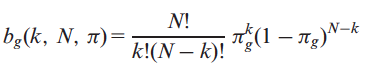where, πg is the probability of having at let one putative deleterious variant (PDV) on the gene g. Therefore, the probability Pg of observing x mutated samples where gene g is potentially impaired and with x≥k is as follows: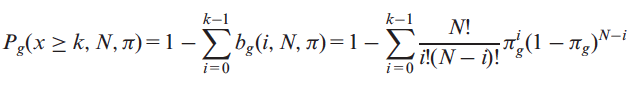where k>0. Using this modified version of the cumulative distribution, we can estimate the probability that a gene g is k or more times classified as a PIG on our dataset. The missing variable for the estimation of Pg is the probability of having at least one PDV in gene g (πg). In our approach, we derived this parameter from the analysis of the occurrence of PIGs in TCGA normal and 1000 Genomes samples. Assuming that rare PDVs have strong functional impact with respect to other types of variants, we classify a gene as a PIG only if it contains at least one PDV with MAF≤0.5% in 1000 Genomes (Khurana, et al., Science, 2013). Therefore, given a set of samples I={I1,I2,..IN}, where N is the total number of samples, the probability πg can be estimated by calculating the putative defective rate (PDR) of the gene g in the dataset I composed by N samples. In our analysis, we defined πg as the maximum PDR value for the gene g in TCGA normal and 1000 Genomes samples. We consider this value as the background PDR of each gene. The πg values described above allow us to calculate the probability Pg that each gene g is classified as a PIG in k or more genomes in a given set of tumor samples. We derive a final score for each gene as follows: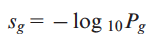In the case where a gene does not harbor any PDV neither in normal TCGA nor in 1000 Genomes samples, an arbitrary PDR of 0.0005 is assigned to this gene. This smoothing of probability is about half of the probability that could be observed by Laplace correction (add one when a value is missing) in 1000 Genomes (1/1,092). Exome scoring method We used the gene scores described above to discriminate between normal and tumor samples. For each genome we extracted the list of M putative impaired genes (PIGs) G={g1,g2,..,gM} with at least one putative deleterious variant (PDV) with allele frequency lower than 0.5% in 1000 Genomes and calculated the average score S as follows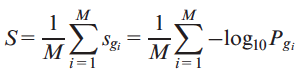where Pg and sg are defined in previous equations. Reference For more details about the methods please refers to the publication reported below and its supplementary material. Tian R, Basu MK, Capriotti E. (2014). ContrastRank: a new method for ranking putative cancer driver genes and classification of tumor samples. Bioinformatics. 30: i572-i578.